84

16

17

12

# GraphRecipes

The repository formerly known as PlotRecipes### Primary author: Thomas Breloff (@tbreloff)

This repo maintains a collection of recipes for graph analysis, and is a reduced and refactored version of the previous PlotRecipes. It uses the powerful machinery of Plots and RecipesBase to turn simple transformations into flexible visualizations.

# Examples

``````using GraphRecipes
using Plots

const n = 15
const A = Float64[ rand() < 0.5 ? 0 : rand() for i=1:n, j=1:n]
for i=1:n
A[i, 1:i-1] = A[1:i-1, i]
end

graphplot(A,
markersize = 0.2,
node_weights = 1:n,
markercolor = range(colorant"yellow", stop=colorant"red", length=n),
names = 1:n,
fontsize = 10,
linecolor = :darkgrey
)
``````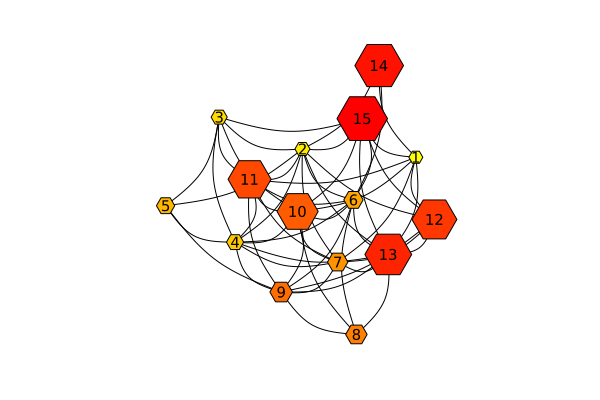Now plot the graph in three dimensions.

``````graphplot(A,
node_weights = 1:n,
markercolor = :darkgray,
dim = 3,
markersize = 5,
linecolor = :darkgrey,
linealpha = 0.5
)
``````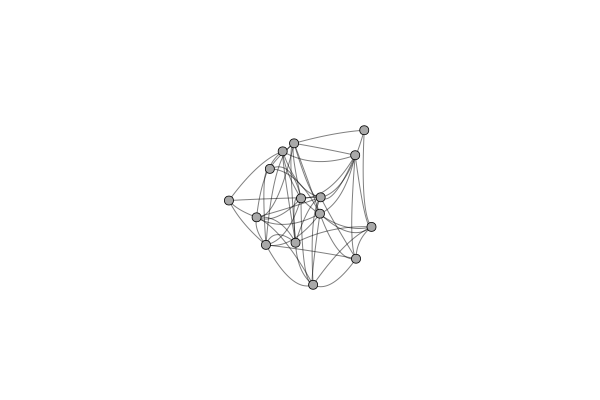### LightGraphs.jl

You can visualize a `LightGraphs.AbstractGraph` by passing it to `graphplot`.

``````using GraphRecipes, Plots
using LightGraphs

g = wheel_graph(10)
graphplot(g, curves=false)
``````#### Directed Graphs -- Only on master!

I you pass `graphplot` a `LightGraphs.DiGraph` or an asymmetric adjacency matrix, then `graphplot` will use arrows to indicate the direction of the edges. Note that using the `arrow` attribute with the `pyplot` backend will allow you to control the aesthetics of the arrows.

``````using GraphRecipes, Plots
g = [0 1 1;
0 0 1;
0 1 0]

graphplot(g, names=1:3, curvature_scalar=0.1)
``````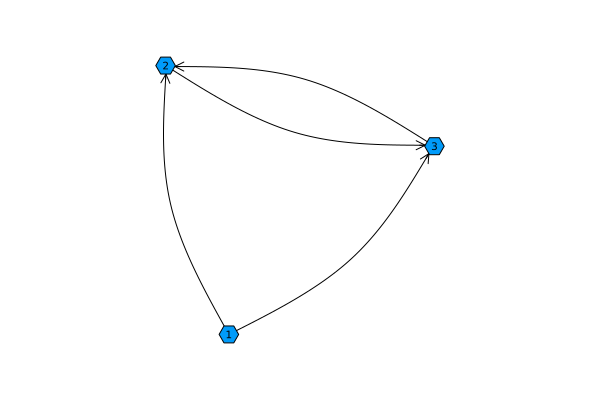#### Edge Labels -- Only on master!

Edge labels can be passed via the `edgelabel` keyword argument. You can pass edge labels as a dictionary of `(si::Int, di::Int) => label`, where `si`, `di` are the indices of the source and destiny nodes for the edge being labeled. Alternatively, you can pass a matrix or a vector of labels. `graphplot` will try to convert any label you pass it into a string unless you pass one of `missing`, `NaN`, `nothing`, `false` or `""`, in which case, `graphplot` will skip the label.

``````using GraphRecipes, Plots
using LightGraphs

n = 8
g = wheel_digraph(n)
edgelabel_dict = Dict()
edgelabel_mat = Array{String}(undef, n, n)
for i in 1:n
for j in 1:n
edgelabel_mat[i, j] = edgelabel_dict[(i, j)] = string("edge ", i, " to ", j)
end
end
edgelabel_vec = edgelabel_mat[:]

graphplot(g, names=1:n, edgelabel=edgelabel_dict, curves=false, nodeshape=:rect)  # Or edgelabel=edgelabel_mat, or edgelabel=edgelabel_vec.
``````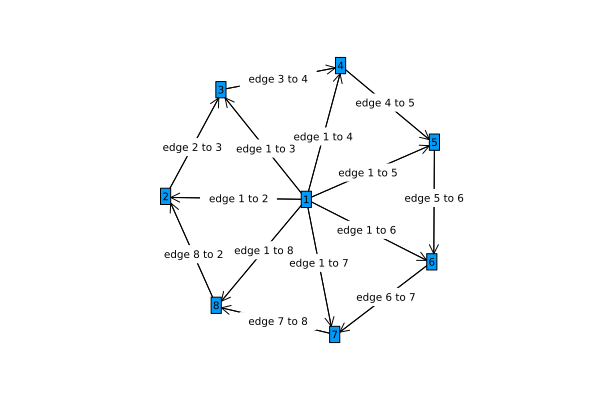#### Self edges -- Only on master!

``````using LightGraphs, Plots, GraphRecipes

g = [1 1 1;
0 0 1;
0 0 1]

graphplot(DiGraph(g), self_edge_size=0.2)
``````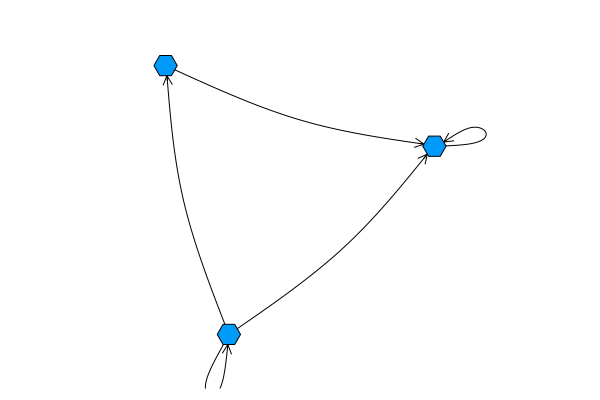#### Multigraphs -- Only on master!

``````graphplot([[1,1,2,2],[1,1,1],], names="node_".*string.(1:3), nodeshape=:circle, self_edge_size=0.25)
``````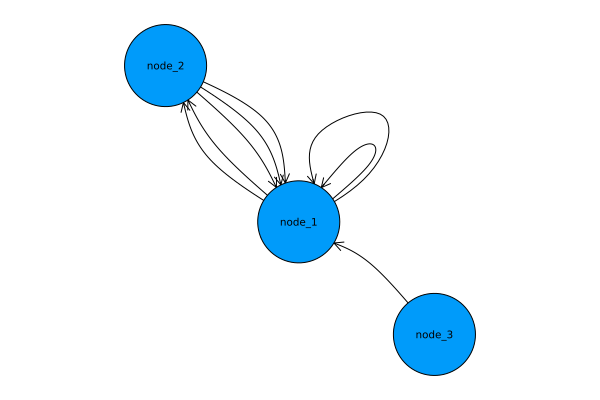#### Arc and chord diagrams

``````using LinearAlgebra
using SparseArrays
using GraphRecipes
using Plots

plot(
method=:chorddiagram,
names=[text(string(i), 8) for i in 1:8],
linecolor=:black,
fillcolor=:lightgray),

method=:arcdiagram,
markersize=0.5,
linecolor=:black,
markercolor=:black)
)
``````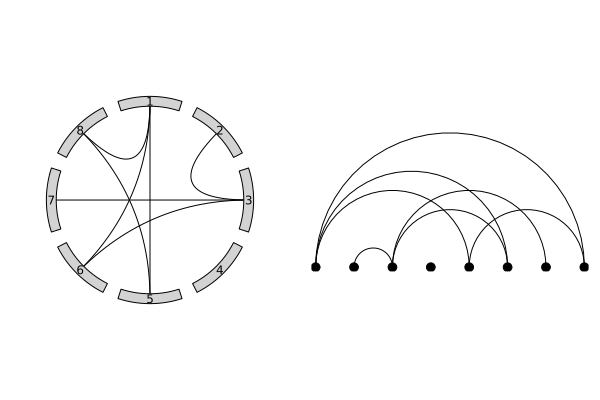#### Julia code -- AST

``````using GraphRecipes
using Plots
default(size=(1000, 1000))

code = :(
function mysum(list)
out = 0
for value in list
out += value
end
out
end
)

plot(code, fontsize=12, shorten=0.01, axis_buffer=0.15, nodeshape=:rect)
``````#### Julia Type Trees -- Only on master!

``````using GraphRecipes
using Plots
default(size=(1000, 1000))

plot(AbstractFloat, method=:tree, fontsize=10, nodeshape=:ellipse)
``````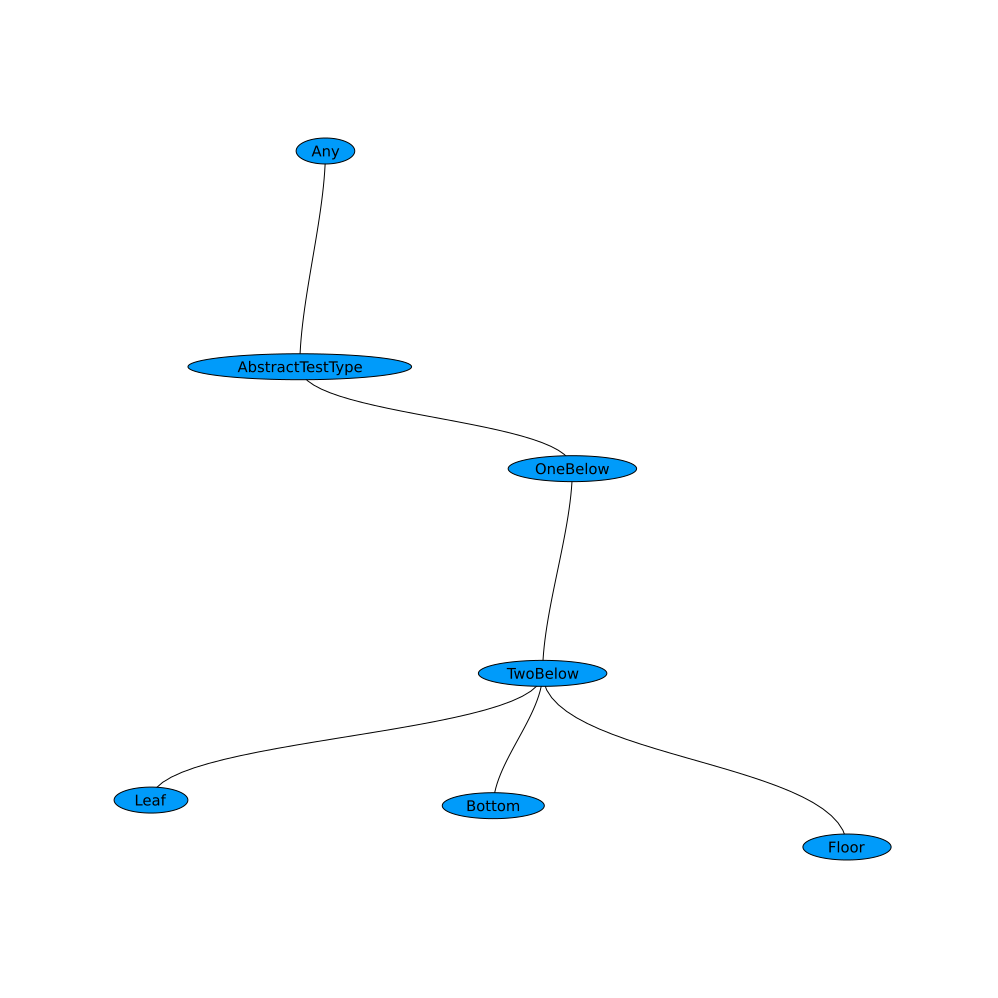#### `AbstractTrees` Trees -- Only on master!

``````using AbstractTrees

AbstractTrees.children(d::Dict) = [p for p in d]
AbstractTrees.children(p::Pair) = AbstractTrees.children(p)
function AbstractTrees.printnode(io::IO, p::Pair)
str = isempty(AbstractTrees.children(p)) ? string(p, ": ", p) : string(p, ": ")
print(io, str)
end

d = Dict(:a => 2,:d => Dict(:b => 4,:c => "Hello"),:e => 5.0)

using GraphRecipes
using Plots
default(size=(1000, 1000))

plot(TreePlot(d), method=:tree, fontsize=10, nodeshape=:ellipse)
``````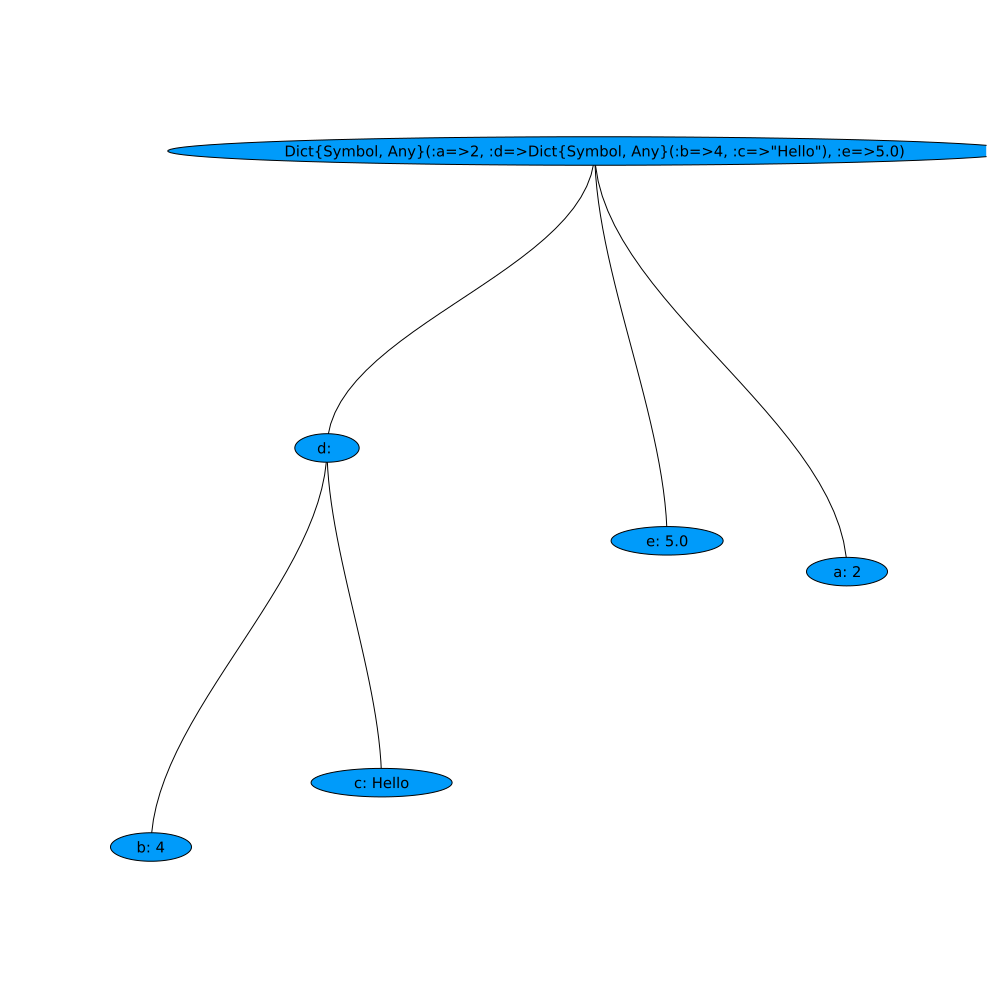05/07/2016

13 days ago

160 commits